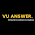# CS201 Assignment 1 Solution 2021 - Introduction to Programming Assignment

CS201 Assignment 1 Solution 2021 - Get Correct CS201 Assignment Solution 2021. CS201 Introduction to Programming Assignment.

Check Here:

CS201 ASSIGNMENT 1 SOLUTION

SPRING 2021

// WELCOME to vuanswer.com the program is written by Sarim

// Don't worry if you need New changed CS201 Assignment free    // my WhatsApp +923162965677

WHATSAPP GROUP JOIN

#include <iostream>

using namespace std;

int main()

{

cout<<"     ---CS201 ASSIGNMENT 1 SOLUTION 1 SPRING 2021----"<<endl;

cout<<" "<<endl;

int x,y,Z;

x=2;

y=1;

Z = (x*x)+(2*y*x)-(x/y);

cout<<"After evaluation of given expression the value of z = "<<Z<<endl;

string ID = "BC";

int id2 = 200205672; // remove and enter any student id here (not real)

string name = "Muhammad Sarim"; // enter your name into my name

int lastdigit;

int Gotnumber;

lastdigit = id2 % 10;

cout<<"--Last digit of VU ID is "<<lastdigit<<endl;

Gotnumber = Z + lastdigit;

// using if else condition

string test;

int n = 1;

if ((lastdigit % 2) == 0)

{

test = "even";

cout<<"I got an "<<test<<" number "<<Gotnumber<<endl;

while (n <= Gotnumber)

{

cout<<"Iteration: "<<n<<endl;

cout<<"My name is "<<name<<endl;

n++;

}

}

else

{

test = " odd";

cout<<"I got an "<<test<<" number "<<Gotnumber<<endl;

while(n <= Gotnumber)

{

cout<<"Iteration: "<<n<<endl;

cout<<"My VU ID is "<<id2<<endl;

n++;

}

}

return 0;

}

CHECK ASSIGNMENT CODE RESULT BELOW

Free assignment keep visit

RESULTMake sure you can make some changes to your solution file before submitting

If you found any mistake then correct yourself

Before submitting an assignment check your assignment requirement file

Comment below if you need some help and question about file and solutions

Sharing with friends

CS201 Assignment 1 Solution Spring 2021

CS201 Introduction to Programming Assignment 1 Solution

Correct CS201 Assignment solution

1.Thank you for cs201 Assignment it's helpful for us

1.Thankyou, keep visiting for study freely.

2.1.2.